Rubik's Magic Cube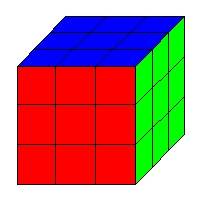Rubik's Cube

In the early 1980's a puzzle invented by Ernö Rubik of Hungary captivated the world's imagination.  Millions of the puzzles were sold, television programs appeared devoted to it and the medical world added "Rubik's thumb," --a repetitive stress, overuse injury from constant twiddling of the cube--to its jargon.

The cube appears to be a 3 x 3 x 3 "stack" of smaller cubes (which we will call cubelets) with an ingenious mechanical design that permits any of the 6 faces of the cube to be rotated.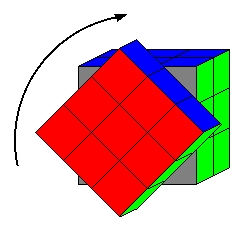Turning the front face

After a succession of such face rotations the cube can become quite mixed up.  The basic problem of the puzzle is to restore the cube to its original condition with all the faces of the cube the same color as shown at the top of this page.  In analyzing the cube we find that the "little cubes" that it is made of, cubelets, are of three varieties.  The cubelets that are in the center of a face, center cubelets, are not moved by a rotation of that, or any, face.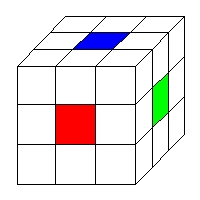Center Cubelets

The remaining cubelets are either edge cubelets which have two colors showing: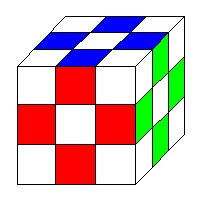Edge cubelets

or corner cubelets which have three colors showing: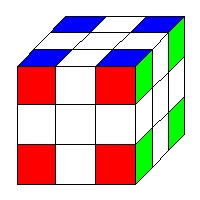Corner cubelets

The first step in analyzing the cube is to devise a notation.  The standard notation consists of naming the faces of the cube according to its orientation in space (with respect to you).  The obvious starting point is to name the faces of the cube by the colors of that face (or at least by the color of the corner cublet which never chianges its position relative to the rest of the cube).  This scheme has the fatal problem that there is no standard way which the faces of the cube are colored.  Therefore we will be using the standard notation based on the cubes orientation.

With the cube on the table in front of you name the face of the cube that is facing you the "Front," call the face opposite the front the "Back;" the face on the right side of the cube is called the "Right;" The face on the left side is the "Left;" the fact pointing upwards is called the "Up" face and the face on the bottom (the one in contact with the table) is the "Down" face.

This naming has the advantage that each face's name begins with a different letter.  Thus we can abbreviate each face with one letter--F, B, R, L, U, D-- without confusion.  Furthermore we will use the abbreviation for each face to symbolize the process of rotating that face.  Thus in the illustraton above the Front face is being rotated clockwise (as viewed when looking at the face) by 90°.  This move is symbolized by "F."  A "kinesthetic" description of the moves F, B, R, L, U and D would be to grasp the cube in the left hand, grasp the face in question with the right hand and turn the named face in the direction pointed to by the right thumb.  This will result in a clockwise rotation viewed as looking at the face.

The opposite or inverse of F for instance would be to turn the front face counter-clockwise 90° -- the reverse of F.  This will be symbolized as F-1 which is the same notation used for group inverses.  Similarly B-1, R-1, L-1, U-1 and D-1 are the inverses of B R L U and D.

With this bit of notation out of the way we can begin to discuss some of the mathematics of the cube.  For instance we notice that any move permutes four corner cubelets and four edge cubelets.  In fact the four corner cubelets perform a four cycle as do the four edge cubelets.  The Up-Front-Left (ufl)corner goes to the place formerly occupied by the Up-Front-Right (ufr) cubelet;  the Up-Front-Right cubelet goes to the place formerly occupied by the Down-Front-Right (dfr) cubelet; the Down-Front-Right cubelet goes to the place formerly occupied by the Down-Front-Left (dfl) cubelet and the Down-Front-Left cubelet completes the four-cycle by moving to the place formerly occupied by the Up-Front-Left cubelet.  We will symbolize this cyclic permutation of the four corner cubelets by

(ufl, rfu, dfr, lfd)

Notice also the order of letters in each three-letter symbol.  The fact that ufl is followed by rfu (rather than by, say, ufr) denotes the fact that

• The upper facelet of the ufr cubelet (u) goes to the right facelet (r) of the rfu cubelet.
• The front facelet of the ufr cubelet (f) goes to the front facelet (f) of the rfu cubelet.
• The left facelet of the ufr cubelet (l) goes to the upper facelet (u) of the rfu cubelet.
[notice the use of lower-case letters for the facelets and cubelets and upper-case letters for the moves and faces of the cube.]

The move F then symbolizes not only a four-cycle of four corner cubelets and a four-cycle of four edge cubelets, but also three four-cycles of the facelets of the four corner cubelets and two four-cycles of the facelets of the edge cubelets.  This leads to some interesting conclusions about what is and isn't possible with the cube.

If we consider only the cubelets (and ignore, for the moment, the facelets of the cubelets) then every move consists of two disjoint four-cycles: one of corner cubelets and one of edge cubelets.  Each of these four-cycles is an odd permutation as we have seen in the last chapter.  Now let's consider the system of corner cubelets and the system of edge cubelets as two separate systems.  Any sequence of moves that has the effect of switching two corner cubelets would be equivalent (as far as the corner cubelets are concerned) with a transposition of two corner cubelets--an odd permutation.  Thus any such process would have to contain an odd number of moves.  This has to follow since every move is an odd permutation and an odd permutation followed by an odd permutation is an even permutation.  Now what happens to the edge cubelets when this hypothetical sequence of moves that transposes two corners is being performed.  More specifically we want to know if such a process can leave the corner cubelets unmolested from their original places.  This would amount to the identity permutation (an even permutation) operating on the system of edge cubelets.  But any process with an odd numbers of moves cannot represent an even permutation of the edge cubelets since every move is a four-cycle (an odd permutation) of edge cubelets.  Thus we have arrived at our first cube theorem:

Theorem: There is no process whose only effect is to switch two corner cubelets.

If we run through the reasoning that led to this result but reverse the roles of corner cubelets and edge cubelets we have a dual of the above theorem:

Theorem: There is no process whose only effect is to switch two edge cubelets.

Thus if one were to come up with a process whose only effect on the corner cubelets is to switch two of them then it would have to perform an odd permutation on the edge cubelets (and vice versa for the dual result).

Both of these theorems are corollaries of a more basic theorem.  Since every move is equivalent to the product of two disjoint four-cycles it is the product of two odd permutations and is therefore an even permutation.  Thus:

Theorem: The only possible processes on the cube are those whose effect is an even permutation of the cubelets.

This limits the possible reconstructions of the cube if it is mechanically disassembled.  If a disassembled cube is reassembled with an odd permutation of the cubelets from the solved state then it will be impossible to solve the cube without resorting to cheating.

If we look more deeply at effect of a move on the cube and consider the facelets of each cubelet then things become a bit more involved.  With this viewpoint a move consists of five disjoint four-cycles.  There are three four-cycles of corner facelets (three on each corner cubelet) and two four-cycles of edge facelets (two on each edge cubelet).  This means that every move is an odd permutation of corner facelets but an even permutation of edge facelets.

From this we can conclude that (1) there is no process that can create an odd permutation of the edge facelets and that (2) an odd permutation of the corner facelets requires an odd number of moves and an even permutation of the corner facelets requires an even number of moves.
From this it follows that

Theorem: There is no process whose only effect on the edge cubelets is to flip one edge cubelet.

Where "flip" refers to having the facelets exchange places.  Note that this theorem says nothing about what happens to the corner cubelets.  It says that if you consider only the effect on the edge cubelets there is no way to flip exactly one of them without disturbing some of the other edge cubelets.  In fact we can extend this theorem to say that there is no process that will flip exactly an odd number of edge cubelets without disturbing any of the other edge cubelets.

With corner cubelets it is not a matter of flipping them as it is twisting them.  A corner cubelet can have three different orientations in any given corner.  We'll study the parity of the corner cubelets using integers mod 3 rather than mod 2 (even / odd) that we used on the edge cubelets.

Grasp a corner cubelet with the thumb and first two fingers of the right hand and imagine turning  it as one turns a screwdriver when driving a right-handed screw.  This will be the positive direction.  The opposite direction will be called the negative direction.  To understand the parity of corner cubelets we'll need to look at the cube in a slightly different light.  Imagine a cube that is white on all its faces except the Up face and the Down face which are colored in.  Below are two illustrations of this cube with (1) a corner cubelet twisted in the negative direction and (2) a corner cubelet twisted in the positive direction.  Since any corner cubelet must be part of either the Up face or the Down face we can look at any corner cubelet at any time and decide whether it has +1 twist, -1 twist or 0 twist.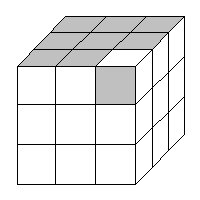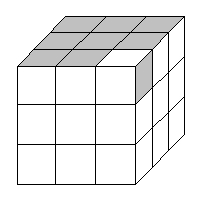(1) Corner with twist -1 (2) Corner with twist +1

Now a -1 twist followed by a +1 twist is the same as 0 twist which is also the same as +3 twist (the identity twist?).  Consider how a move such as F affects the twist parity of the corner cubelets.  If F is applied to a cube the ufl cubelet goes to the rfu position.  In doing so it now has been given a +1 twist.  It appears as the corner cubelet in illustration (2) with respect to the UP face.  Similarly the dfl cubelet will become the lfu cubelet and will now have been given a -1 twist.  Thus the twists on the same side (L in this case) will cancel each other out so the total twist parity due to these two cubelets is 0.  The same thing will happen with the corner cubelets on the right front.  One will be given a +1 twist and the other will be given a -1 twist.

So a single move cannot change the total twist parity of the corners it affects.  Also for this simplified cube (with only the top and bottom colored) any turn of the Up or Down faces doesn't change the parity of any cubelets.  We can conclude that

Theorem: The total twist parity of the cube cannot change.

So what kinds of twists of the corners can be acheived with the cube?  Well, it's possible to twist two corners in opposite directions wiht  0ne getting a +1 twist and the other a -1 twist for a total twist parity change of 0.  It is also possible to twist three corner cubelets in the same direction either each +1 or each -1 which still gives a total twist parity change of 0 (1 + 1 + 1 = 0 mod 3).

Putting all these results together we find that if we were to disassemble a cube we would have some significant choices on how to reassemble it.  (1) we could either reassemble it with an edge cubelet flipped or not and (2) we could reassemble it with a corner cubelet given a +1 twist, a -1 twist or a zero twist and finally (3) we could reassemble it with a pair of corner cubelets exchanged [or instead a pair of edge cubelets exchanged].  There are two independent ways do (1).  Either we flip an edge or not.  There are three independent ways to do (2): +1, -1 or 0. [It doesn't matter which corner we give the +1 twist to, we can transfer that +1 twist to another corner by cube processes later so all reassemblings with a +1 corner twist given to some corner are equivalent]  and finally there are two ways to do (3):  either switch two cubelets or not.  [again which two cubelets we switch doesn't matter since we can use cube processes to go from any two switched edge cubelets to any two other switched edge cubelets and the same for switched corner cubelets].

Putting it all together we find that there are 12 (= 2 x 3 x 2) independent ways to reassemble the cube.  Thus if we were to take a cube apart and reassemble it in the dark we would have a 1 out of 12 chance of having a solvable cube.

Send comments, corrections and criticisms to:

dogschool@dog.com